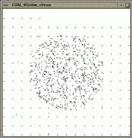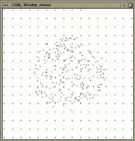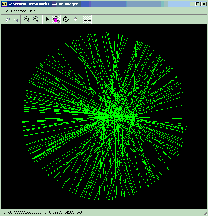# Chapter 58Geometric Object Generators

Susan Hert, Michael Hoffmann, Lutz Kettner, and Sven Schönherr

 58.1 Introduction 58.1.1   Random Perturbations 58.1.2   Adding Degeneracies 58.1.3   Support Functions and Classes for Generators 58.2 Example Generating Degenerate Point Sets 58.3 Example Generating Grid Points 58.4 Examples Generating Segments

## 58.1   Introduction

A variety of generators for geometric objects are provided in CGAL. They are useful as synthetic test data sets, e.g. for testing algorithms on degenerate object sets and for performance analysis.

Two kinds of point generators are provided: first, random point generators and second deterministic point generators. Most random point generators and a few deterministic point generators are provided as input iterators. The input iterators model an infinite sequence of points. The function CGAL::copy_n() can be used to copy a finite sequence; see Section 55.6. The iterator adaptor Counting_iterator can be used to create finite iterator ranges; see Section 55.6. Other generators are provided as functions that write to output iterators. Further functions add degeneracies or random perturbations.

In 2D, we provide input iterators to generate random points in a disc (Random_points_in_disc_2), in a square (Random_points_in_square_2), on a circle (Random_points_on_circle_2), on a segment (Random_points_on_segment), and on a square (Random_points_on_square_2). For generating grid points we provide three functions, points_on_segment_2, points_on_square_grid_2 that write to output iterators and an input iterator Points_on_segment_2.

For 3D points, input iterators are provided for random points uniformly distributed in a sphere (Random_points_in_sphere_3) or cube (Random_points_in_cube_3) or on the boundary of a sphere (Random_points_on_sphere_3). For generating 3D grid points, we provide the function points_on_cube_grid_3 that writes to an output iterator.

We also provide two functions for generating more complex geometric objects. The function random_convex_set_2 computes a random convex planar point set of a given size where the points are drawn from a specific domain and random_polygon_2 generates a random simple polygon from points drawn from a specific domain.

### 58.1.1   Random Perturbations

Degenerate input sets like grid points can be randomly perturbed by a small amount to produce quasi-degenerate test sets. This challenges numerical stability of algorithms using inexact arithmetic and exact predicates to compute the sign of expressions slightly off from zero. For this the function perturb_points_2 is provided.

For a given point set certain kinds of degeneracies can be produced by adding new points. The random_selection() function is useful for generating multiple copies of identical points. The function random_collinear_points_2() adds collinearities to a point set.

### 58.1.3   Support Functions and Classes for Generators

The function random_selection chooses n items at random from a random access iterator range which is useful to produce degenerate input data sets with multiple entries of identical items.

## 58.2   Example Generating Degenerate Point Sets

We want to generate a test set of 1000 points, where 60% are chosen randomly in a small disc, 20% are from a larger grid, 10% are duplicates points, and 10% collinear points. A random shuffle removes the construction order from the test set. See Figurefor the example output.

```File: examples/Generator/random_degenerate_point_set.cpp
```
```#include <CGAL/Simple_cartesian.h>
#include <cassert>
#include <vector>
#include <algorithm>
#include <CGAL/point_generators_2.h>
#include <CGAL/copy_n.h>
#include <CGAL/random_selection.h>

using namespace CGAL;

typedef Simple_cartesian<double>         R;
typedef R::Point_2                       Point;
typedef Creator_uniform_2<double,Point>  Creator;
typedef std::vector<Point>               Vector;

int main() {
// Create test point set. Prepare a vector for 1000 points.
Vector points;
points.reserve(1000);

// Create 600 points within a disc of radius 150.
Random_points_in_disc_2<Point,Creator> g( 150.0);
CGAL::copy_n( g, 600, std::back_inserter(points));

// Create 200 points from a 15 x 15 grid.
points_on_square_grid_2( 250.0, 200, std::back_inserter(points),Creator());

// Select 100 points randomly and append them at the end of
// the current vector of points.
random_selection( points.begin(), points.end(), 100,
std::back_inserter(points));

// Create 100 points that are collinear to two randomly chosen
// points and append them to the current vector of points.
random_collinear_points_2( points.begin(), points.end(), 100,
std::back_inserter( points));

// Check that we have really created 1000 points.
assert( points.size() == 1000);

// Use a random permutation to hide the creation history
// of the point set.
std::random_shuffle( points.begin(), points.end(), default_random);

// Check range of values.
for ( Vector::iterator i = points.begin(); i != points.end(); i++){
assert( i->x() <=  251);
assert( i->x() >= -251);
assert( i->y() <=  251);
assert( i->y() >= -251);
}
return 0;
}
```

 Figure: Output of example program for point generators.## 58.3   Example Generating Grid Points

The second example demonstrates the point generators with integer points. Arithmetic with doubles is sufficient to produce regular integer grids. See Figurefor the example output.

```File: examples/Generator/random_grid.cpp
```
```#include <CGAL/Simple_cartesian.h>
#include <cassert>
#include <vector>
#include <algorithm>
#include <CGAL/point_generators_2.h>
#include <CGAL/copy_n.h>

using namespace CGAL;

typedef Simple_cartesian<int>         R;
typedef R::Point_2                    Point;
typedef Creator_uniform_2<int,Point>  Creator;

int main() {
// Create test point set. Prepare a vector for 400 points.
std::vector<Point> points;
points.reserve(400);

// Create 250 points from a 16 x 16 grid. Note that the double
// arithmetic _is_ sufficient to produce exact integer grid points.
// The distance between neighbors is 34 pixel = 510 / 15.
points_on_square_grid_2( 255.0, 250, std::back_inserter(points),Creator());

// Lower, left corner.
assert( points.x() == -255);
assert( points.y() == -255);

// Upper, right corner. Note that 6 points are missing to fill the grid.
assert( points.x() == 255 - 6 * 34);
assert( points.y() == 255);

// Create 250 points within a disc of radius 150.
Random_points_in_disc_2<Point,Creator> g( 150.0);
CGAL::copy_n( g, 250, std::back_inserter(points));

// Check that we have really created 500 points.
assert( points.size() == 500);
return 0;
}
```

 Figure: Output of example program for point generators working on integer points.## 58.4   Examples Generating Segments

The following two examples illustrate the use of the generic functions from Section 55.6 like Join_input_iterator_2 to generate composed objects from other generators - here two-dimensional segments from two point generators.

We want to generate a test set of 200 segments, where one endpoint is chosen randomly from a horizontal segment of length 200, and the other endpoint is chosen randomly from a circle of radius 250. See Figurefor the example output.

```File: examples/Generator/random_segments1.cpp
```
```#include <CGAL/Simple_cartesian.h>
#include <cassert>
#include <vector>
#include <algorithm>
#include <CGAL/Point_2.h>
#include <CGAL/Segment_2.h>
#include <CGAL/point_generators_2.h>
#include <CGAL/function_objects.h>
#include <CGAL/Join_input_iterator.h>
#include <CGAL/copy_n.h>

using namespace CGAL;

typedef Simple_cartesian<double>         R;
typedef R::Point_2                       Point;
typedef Creator_uniform_2<double,Point>  Pt_creator;
typedef R::Segment_2                     Segment;
typedef std::vector<Segment>             Vector;

int main() {
// Create test segment set. Prepare a vector for 200 segments.
Vector segs;
segs.reserve(200);

// Prepare point generator for the horizontal segment, length 200.
typedef  Random_points_on_segment_2<Point,Pt_creator>  P1;
P1 p1( Point(-100,0), Point(100,0));

// Prepare point generator for random points on circle, radius 250.
typedef  Random_points_on_circle_2<Point,Pt_creator>  P2;
P2 p2( 250);

// Create 200 segments.
typedef Creator_uniform_2< Point, Segment> Seg_creator;
typedef Join_input_iterator_2< P1, P2, Seg_creator> Seg_iterator;
Seg_iterator g( p1, p2);
CGAL::copy_n( g, 200, std::back_inserter(segs));

assert( segs.size() == 200);
for ( Vector::iterator i = segs.begin(); i != segs.end(); i++){
assert( i->source().x() <=  100);
assert( i->source().x() >= -100);
assert( i->source().y() ==    0);
assert( i->target().x() * i->target().x() +
i->target().y() * i->target().y() <=  251*251);
assert( i->target().x() * i->target().x() +
i->target().y() * i->target().y() >=  249*249);
}
return 0;
}
```

 Figure: Output of example program for the generic segment generator.The second example generates a regular structure of 100 segments; see Figurefor the example output. It uses the Points_on_segment_2 iterator, Join_input_iterator_2 and Counting_iterator to avoid any intermediate storage of the generated objects until they are used, which in this example means copied to a window stream.

```File: examples/Generator/random_segments2.cpp
```
```#include <CGAL/Simple_cartesian.h>
#include <algorithm>
#include <vector>
#include <CGAL/point_generators_2.h>
#include <CGAL/function_objects.h>
#include <CGAL/Join_input_iterator.h>
#include <CGAL/Counting_iterator.h>

using namespace CGAL;

typedef Simple_cartesian<double>                  R;
typedef R::Point_2                                Point;
typedef R::Segment_2                              Segment;
typedef Points_on_segment_2<Point>                PG;
typedef Creator_uniform_2< Point, Segment>        Creator;
typedef Join_input_iterator_2< PG, PG, Creator>   Segm_iterator;
typedef Counting_iterator<Segm_iterator,Segment>  Count_iterator;
typedef std::vector<Segment>                      Vector;

int main() {
// Create test segment set. Prepare a vector for 100 segments.
Vector segs;
segs.reserve(100);

// A horizontal like fan.
PG p1( Point(-250, -50), Point(-250, 50),50);   // Point generator.
PG p2( Point( 250,-250), Point( 250,250),50);
Segm_iterator  t1( p1, p2);                     // Segment generator.
Count_iterator t1_begin( t1);                   // Finite range.
Count_iterator t1_end( 50);
std::copy( t1_begin, t1_end, std::back_inserter(segs));

// A vertical like fan.
PG p3( Point( -50,-250), Point(  50,-250),50);
PG p4( Point(-250, 250), Point( 250, 250),50);
Segm_iterator  t2( p3, p4);
Count_iterator t2_begin( t2);
Count_iterator t2_end( 50);
std::copy( t2_begin, t2_end, std::back_inserter(segs));

CGAL_assertion( segs.size() == 100);
for ( Vector::iterator i = segs.begin(); i != segs.end(); i++){
CGAL_assertion( i->source().x() <=  250);
CGAL_assertion( i->source().x() >= -250);
CGAL_assertion( i->source().y() <=  250);
CGAL_assertion( i->source().y() >= -250);
CGAL_assertion( i->target().x() <=  250);
CGAL_assertion( i->target().x() >= -250);
CGAL_assertion( i->target().y() <=  250);
CGAL_assertion( i->target().y() >= -250);
}
return 0;
}
```

 Figure: Output of example program for the generic segment generator using pre-computed point locations.